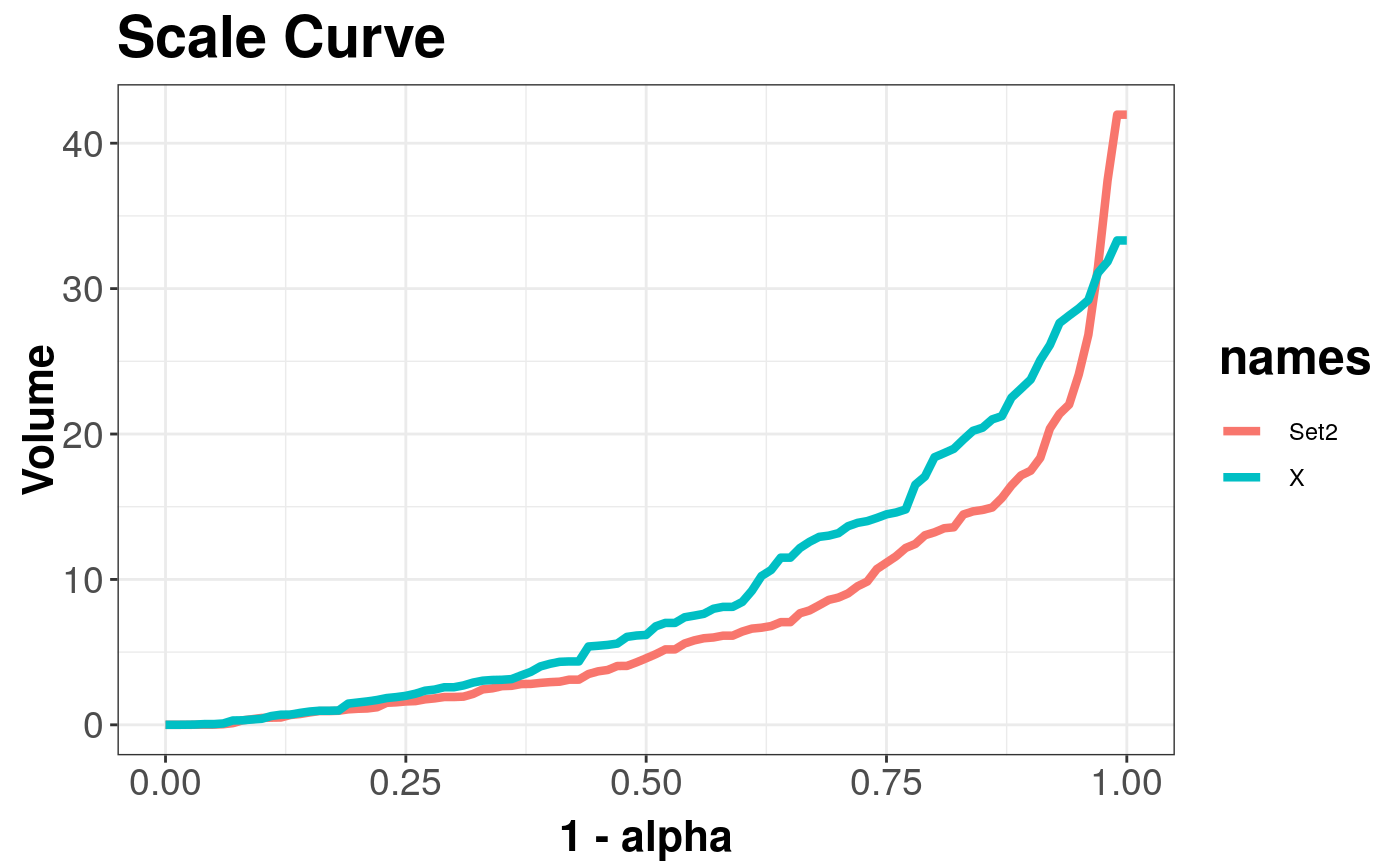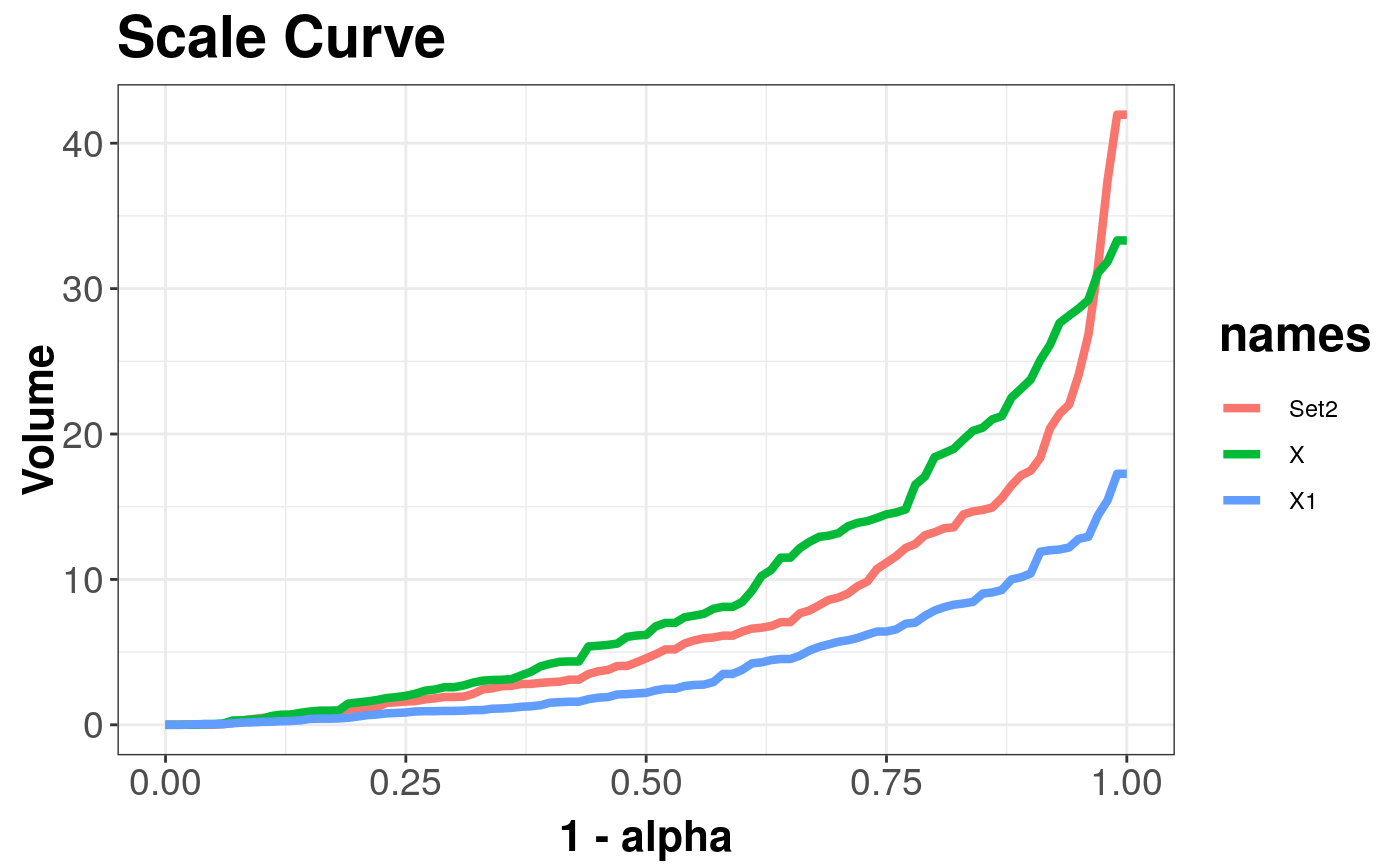## Details

DepthCurve is a virtual class that contains methods (getPlot(...) and plot(...)) for rendering single curve such as ScaleCurve or AsymmetryCurve. Such object can be combined by overloaded operator '

## Slots

depth

object of Depth-class

name

name of dataset used on plot

title

title of a plot

alpha

central area values

## Examples

library(mvtnorm)
x <- mvrnorm(n = 100, mu = c(0, 0), Sigma = 2 * diag(2))
y <- rmvt(n = 100, sigma = diag(2), df = 4)
s1 <- scaleCurve(x, depth_params = list(method = "Projection"))
s2 <- scaleCurve(y, depth_params = list(method = "Projection"), name = "Set2")

sc_list <- combineDepthCurves(s1, s2) # Add one curve to another

plot(sc_list) # Draw plot with two curvesz <- mvrnorm(n = 100, mu = c(0, 0), Sigma = 1 * diag(2))
s3 <- scaleCurve(z, depth_params = list(method = "Projection"))
plot(combineDepthCurves(sc_list, s3)) # Add third curve and draw a plot#> Warning: Names in DepthCurveList are not unique!